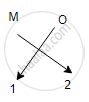# Based on the Group Valency of Element Write the Molecular Formula of the Following Compound Giving Justification: Oxide of First Group Elements. - Science

Short Note

Based on the group valency of element write the molecular formula of the following compound giving justification:
Oxide of first group elements.

#### Solution

Oxides of the first group elements have the common formula of M20.
Example- Na20, K20. This is because, the first group elements have a common valency of 1, and the valency of Oxygen is 2 so, to satisfy the combining capacity of Oxygen two 1st group metals are required.Is there an error in this question or solution?
2018-2019 (March) 31/1/3

Share